## Quaterniones

 Quaternions are similar to complex and hyper complex numbers, but their multiplication is not cummutativeThey have 3 non real parts, that are marked here with the letters j, k and l. The real part will not have an own letter here.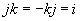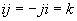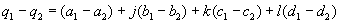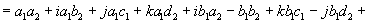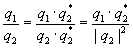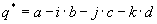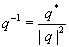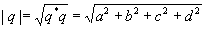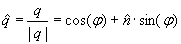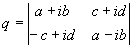### Description of rotationcorresponds to the rotation angle.corresponds to the rotation axis.  Quaternions are mainly used in computer graphics to descibe rotations in the space. Also they are used in quant mechanics to describe spins. Doing this, put instead of the marks (e,i,j,k) the Pauli spin matrices: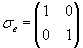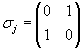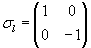### Rotations with quaternions

 A point P=(x,y,z) is represented with the quaternion p = ix + jy + kz .A rotation of the anglearound the x-axis is represented with the quaternion. The rotated point is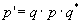.

### Conversion of quaternion to rotation matrix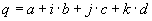-->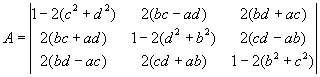### Conversion of rotation matrix to quaternion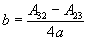d### Trigonometric functions of quaternions

 At the following procedure in a 1st step the quaternions will be converted to complex numbers. Then in a 2nd step the complex funktion is executed. In a 3rd step the complex result will be converted to a quaternion using the old quaternion.Because I didnot find a publication with a proper description about this topic, I dont know whether this is correct and whether I can use this procedure for all function I used it. It would be nice to get a hint about this. (email: calc_3d@greuer.de)

### Procedure

 With the quaternionThe function func(q) should be executedq can be seperated into the
 real part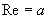und den imaginary part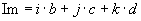With this a complex number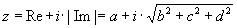is created.The function func(z) is executed with this complex number:Using the imaginary part of the former quaternion the result is created:with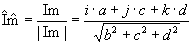This procedure is used in Calc 3D for the following functions:sin; cos; tan; sinh; cosh; tanh; arcsin; arccos; arctan; arccot; arsinh; arcosh; artanh; arcoth; log; exp